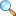# MTH480: Introductory Quantum Mechanics

The physical principles and mathematical formalism of quantum theory, with emphasis on applications to atomic, molecular, and many-body physics; scattering phenomena; and electromagnetism (photon physics).

It is intended to supplement the discussion of hydrogen and many-electron orbital’s commonly found in general chemistry text books with a deeper understanding of the underlying physical principles. The relationship between classical and quantum mechanics is explored to illustrate how physical objects can be viewed both as a particle and a wave.

Basic postulates of quantum mechanics. State vectors. Formal properties of quantum mechanical operators. Eigenvalues and eigenstates, simple harmonic oscillator. Schrodinger representation. Heisenberg equation of motion Schrodinger equation. Potential step, potential barrier, potential well. Orbital angular momentum. Motion in a centrally symmetric field. Hydrogen atom. Matrix representation of angular momentum and spin. Time independent perturbation theory, degeneracy. The Stark effect. Introduction to relativistic Quantum Mechanics.

1. Let $x(t)={{t}^{3}}+2\sin t$ represents some distance function at point t.
1. Find the velocity and acceleration at time t.
2. Find the velocity at time 5sec.
3. Find acceleration at $t=\dfrac{\pi }{6}$ sec.
4. What is the kinetic energy at time 2.5sec if mass is 52kg.
2. Suppose an electric train start its journey from zero to the velocity function $v(t)={{t}^{2}}+t{{e}^{t}}$.
1. Find the distance traveled by the train in KM after 5mints.
2. Find the velocity of train after one hours.
3. Find the momentum of the train just after 5 seconds if weight of the train is 100 tons.

Please download PDF files of the notes given below. To view PDF files, there must be PDF Reader (Viewer) installed on your PC or mobile or smartphone. It can be downloaded from Software section or a user viewing on Android Smartphone may consider EBookDroid to view PDF on their smartphone.

• Assignment 02 | Download PDF |View OnlinePlease click on View Online to see inside the PDF.